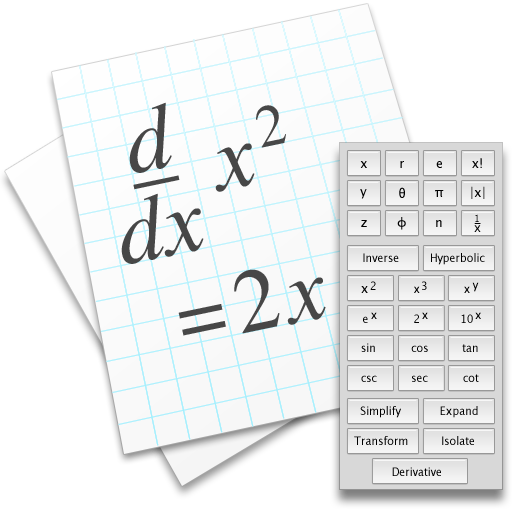Change the way you install and update apps with the all new
MacUpdate Desktop

Thank you for your reviewDeveloper website:

#### Top community discussions about Equation Calculator

• Is there a better alternative to Equation Calculator?

See discussion
• Is Equation Calculator really the best app in Finance category?

See discussion
• Will Equation Calculator work good on macOS 10.13.4?

See discussion
Equation Calculator is a scientific calculator which does symbolic and algebraic manipulation, algebra and calculus as well as numeric computation. You can define variables and functions, evaluate symbolic derivatives, numeric integrals and matrix operations.

### What's New in Equation Calculator

Version 1.1:
• Users can now type text comments in documents.

### Requirements for Equation Calculator

Mac OS X 10.6 or later
Similar Software
Suggest other similar softwareLike this app? Be the first to add it to a collection! Create a Collection by bringing together complementary apps that have a common theme or purpose, then share it and discover new collections!

### Equation Calculator User Discussion

5 1
Ratings
Overall
(1)
Current Version (1.x)
(1)
Details